The whole loaded train weighs 360 tons. It has twenty wagons, each carrying 12 tons of grain. What is the weight of the locomotive?

l =  120 t

### Step-by-step explanation: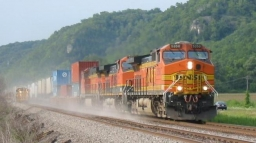Did you find an error or inaccuracy? Feel free to write us. Thank you!## Related math problems and questions:

• Locomotive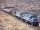The whole loaded train weighs 760 tons. It has twenty wagons, each carrying 20 tons of grain and empty weighs 10 tons. How much weight a locomotive?
• Train tons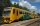The entire loaded train weighs 760 tons. It has a total of 30 wagons, each carrying 12 tons of grain and empty weighs 10 tons. What is the weight of the locomotive?
• Freight train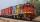Twenty-five identical freight wagons are connected behind the locomotive. The weight of the locomotive and all wagons is 1009000 kg. The locomotive weighs 84 tons. How many kilograms does one freight car weigh?
• The bookkeeperThe bookkeeper bought 45 copies of fairy tale books for CZK 120 and 65 copies of the encyclopedia for CZK 325. then he sold it in his shop like this: fairy tales for 135 CZK and encyclopedias for 360 CZK. What was the purchase price of the books? And the
• Piggy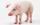A man bought a pig for € 60 then sold it for € 75. Then again bought for € 90 and sold it for € 100. How many € earned?
• Subtraction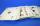How many times you can subtract the number 4 from the number 64?
• Freight train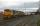The train carries 525 tons of limestone in 29 wagons. Wagons are 15 tonne and 20 tons. How many is 15 ton and how many is 20 ton wagons?
• ConcertOn a Concert were sold 150 tickets for CZK 360, 235 tickets for 240 CZK and 412 for 180 CZK. How much was the total revenues for tickets?
• A farmer's 2A farmer's truck is carrying 4 2/3 tons of hay and straw. If 3 2/5 tons of the load is hay, how much straw is the truck carrying?
• How manzBy how many are the product of the numbers 328 and 7 greater than their sum?
• RemaindersIt is given a set of numbers { 170; 244; 299; 333; 351; 391; 423; 644 }. Divide this numbers by number 66 and determine set of remainders. As result write sum of this remainders.
• Identity123456789 = 100 Use only three plus or minus characters to correct previous identity/equation.Add marks (+, -, *, /, brackets) to fullfill equations 1 3 6 5 = 10 This is for the 4th grade of the primary school - with no negative numbers yet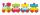The numbers 1,2,3,4,5,6,7,8 and 9 traveled by train. The train had three cars and each was carrying just three numbers. No. 1 rode in the first carriage, and in the last carriage was all odd numbers. The conductor calculated sum of the numbers in the firs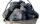Coal hopper has a capacity of 285 liters. How many tons is it? The bulk density of coal is 916 kg/m3.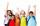How many children are in the classroom, where Ladislav is 10th lightest and 16th heaviest in class?Emil has 23 pencils in a pencil case, 18 of which were broken. Emily 9 trims, 2 breaks, 4 trims and goes to school. When he returned from school, he has broken 5 pencils. How many pencils have trimmed at school?